# CHEM.1230L: Chemistry I Lab

## Experiment 6: Percent Composition of a Carbonate

Overview

"Carbonates" are compounds which contain the carbonate ion, CO32-. In this experiment you will be given a sample of an "unknown" carbonate, and you will determine what percentage (by mass) of carbonate ion the compound contains. Although you will make three determinations of your sample, realize that the percent carbonate calculated for your sample should be the same (within reasonable experimental error) for each of the determinations. If you are not careful in this experiment, or if you hurry the experiment because it seems so "easy" to perform, you will receive poor agreement between your three determinations and you will receive a poor grade on your lab report.

When a compound containing the carbonate ion is treated with an acid, the following net reaction occurs:

2H+(aq) + CO32- (aq)  ®  H2O(l) + CO2(g)

CO2 is a gas and is lost from the carbonate sample when the acid is added to the sample. In this experiment, we determine how much carbonate ion is present in a sample by measuring the loss in mass (which represents the CO2 that escapes) when acid is added to the sample. For example, if a carbonate sample decreases in mass by 0.2571 g when treated with acid, then the sample must have contained the following amount of carbonate ion: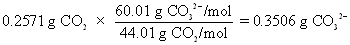The numbers (60.01 g CO32-/mol) and (44.01 g CO2/mol) are the molar masses (formula weights) of the carbonate ion and carbon dioxide, respectively.

You perform this experiment in "microscale" using very small samples. For this purpose, balances are provided which make mass determinations to 0.0001 g (four decimal places). You should carry out all your calculations maintaining this level of precision.

## Calculations

Suppose the following data have been recorded for the analysis of a carbonate sample (Page 27, Part I)

 Trial 1 Trial 2 Trial 3 Mass beaker, tube, HCl 9.7821 g 9.8835 g 9.6642 g Mass beaker, tube, HCl, sample (before rxn) 10.2758 g 10.3792 g 10.1667 g Mass beaker, tube, HCl, sample (after rxn) 10.0536 g 10.1561 g 9.9410 g

## Page 27, Part 2

1. Mass of sample (for Trial 1)

The mass of carbonate sample added to the test tube for Trial 1 will be the difference in mass between the (mass of beaker, tube, HCl, sample before reaction) and the (mass of beaker, tube HCl). Using the data above, calculate the mass of carbonate sample for Trial 1, then click here to check your answer.

2. Mass of CO2 evolved (for Trial 1)

The mass of CO2 evolved represents the change in mass before and after the reaction (i.e., when the acid was transferred from the pipet to the carbonate sample). For the table of data above, the mass of carbon dioxide evolved represents the difference between the (mass of beaker, tube, HCl, sample before reaction) and the (mass of beaker, tube, HCl, sample after reaction). Calculate the mass of CO2 evolved for Trial 1 above, then click here to check your answer.

3. Percent Carbonate in Unknown (for Trial 1)

The actual mass of carbonate in the sample used for Trial 1 must first be calculated, before the percent carbonate can be determined. The sample for Trial 1 evolved 0.2222 g CO2. The mass of carbonate ion that would enable this amount of CO2 to be generated is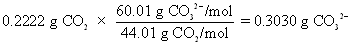The numbers (60.01 g CO32-/mol) and (44.01 g CO2/mol) are the molar masses (formula weights) of the carbonate ion and carbon dioxide, respectively.

The percent carbonate in the sample used in Trial 1 is based on the mass of carbonate ion in the sample (0.3030 g CO32-) and the total mass of the sample before reaction (0.4937 g). Calculate the percent carbonate determined for the sample used in Trial 1 above, then click here to check your answer.

4. Average % carbonate in unknown

This represents the sum of the % carbonates determined for the three trials, divided by the number of trials (three). The three individual percentages determined for the three Trials are shown below, along with the calculation of the average percent carbonate.

 Trial 1 Trial 2 Trial 3 % Carbonate 61.37% CO32- 61.37% CO32- 61.24% CO32-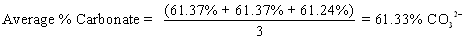5. Deviations in % Carbonate

For Trial 1, the deviation from the average is calculated as

 Deviation Trial 1 = (61.37% - 61.33%) = 0.04% CO32-

The deviations for the three results are summarized below:

 Trial 1 Trial 2 Trial 3 Deviation 0.04 % CO32- 0.04 % CO32- -0.09 % CO32-

6. Average Deviation in % Carbonate

The average deviation is the sum of the absolute values of the individual deviations, divided by the number of trials (three)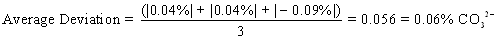7. Summary of results

For the sample data above, we would report the % carbonate in the unknown sample as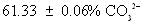## Question

This problem is solved almost exactly the same way you treat the data in your experiment. The data is given in a slightly different format, however, so make sure you know what number to use in what place. The answer is 17.13% carbonate but you will have to show exactly how to get this answer to receive credit for the problem on your lab report.